ENGR338 Lab 2021 Spring
Lab 9 Design a Simple 8-Bit ALU
Name:
Sean Eaton
Email: smeaton@fortlewis.edu

Design a Simple 8-bit ALU

Introduction

In this lab we were tasked with designing an 8-bit arithmetic logic unit (ALU). The simple 8-bit ALU is able to perform addition and subtraction arithmetic operations as well as AND and OR logical operations on two 8-bit inputs. Two selection inputs, S0 and S1, are used to select between the four ALU operations. This lab built upon previous labs that tasked us with designing 8-bit AND and OR gates, the 8-bit high-speed full adder, and the 8-bit 2:1 multiplexer. These schematics, icons, and layouts were used to complete the 8-bit ALU. The logic of the ALU was simulated previous to the layout view being created.

Task 1: Design the logic circuit of the ALU.
The schematic of the ALU was provided to us in the lab instructions and so it was recreated in Electric VLSI using the appropriate designs that were created previously in the lab. Only one additional design had to be created prior to the 8-bit ALU's implementation which was the 8-bit inverter. The 1-bit inverter design was imported and the process used in previous labs was used to create an 8-bit inverter. The finished schematic and icon view are shown below as well as the 8-bit inverter's layout view.Figure 1. Schematic and icon of the 8-bit inverter. The icon of the 1-bit schematic was used with additional bus inputs and outputs.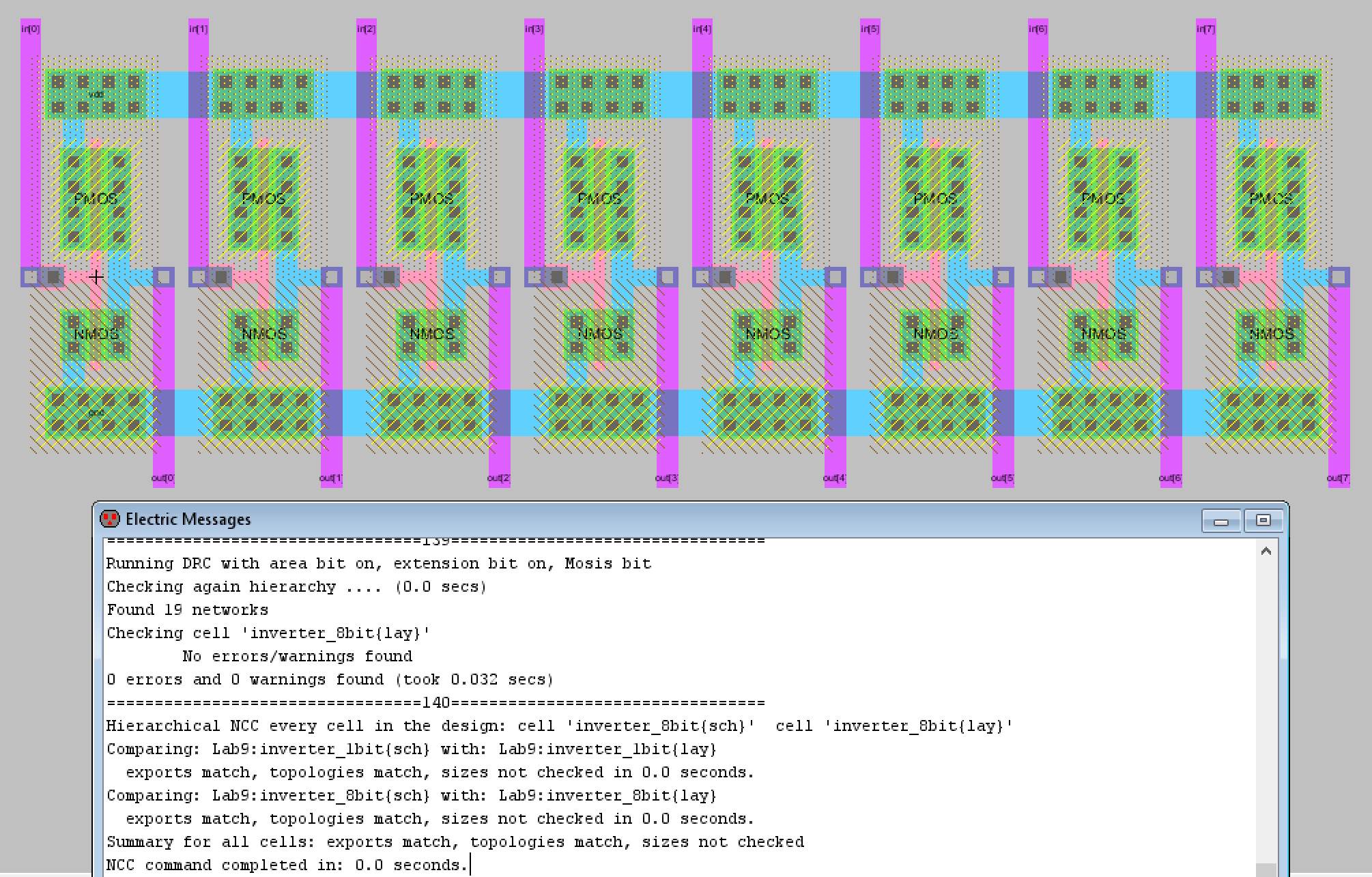Figure 2. Final layout view of the 8-bit inverter. The 1-bit inverter's layout view was used to create the 8-bit inverter's final layout.

Now that an 8-bit inverter was available to use, the schematic of the ALU was recreated in Electric VLSI. The schematic made use of the 8-bit AND gate, OR gate, MUX, inverter, and high-speed full adder. Initially the schematic had the A and B inputs for the 8-bit OR gate reversed but this was fixed once the layout view was completed. The final schematic for the ALU is shown below in Figure 3 along with the icon I created.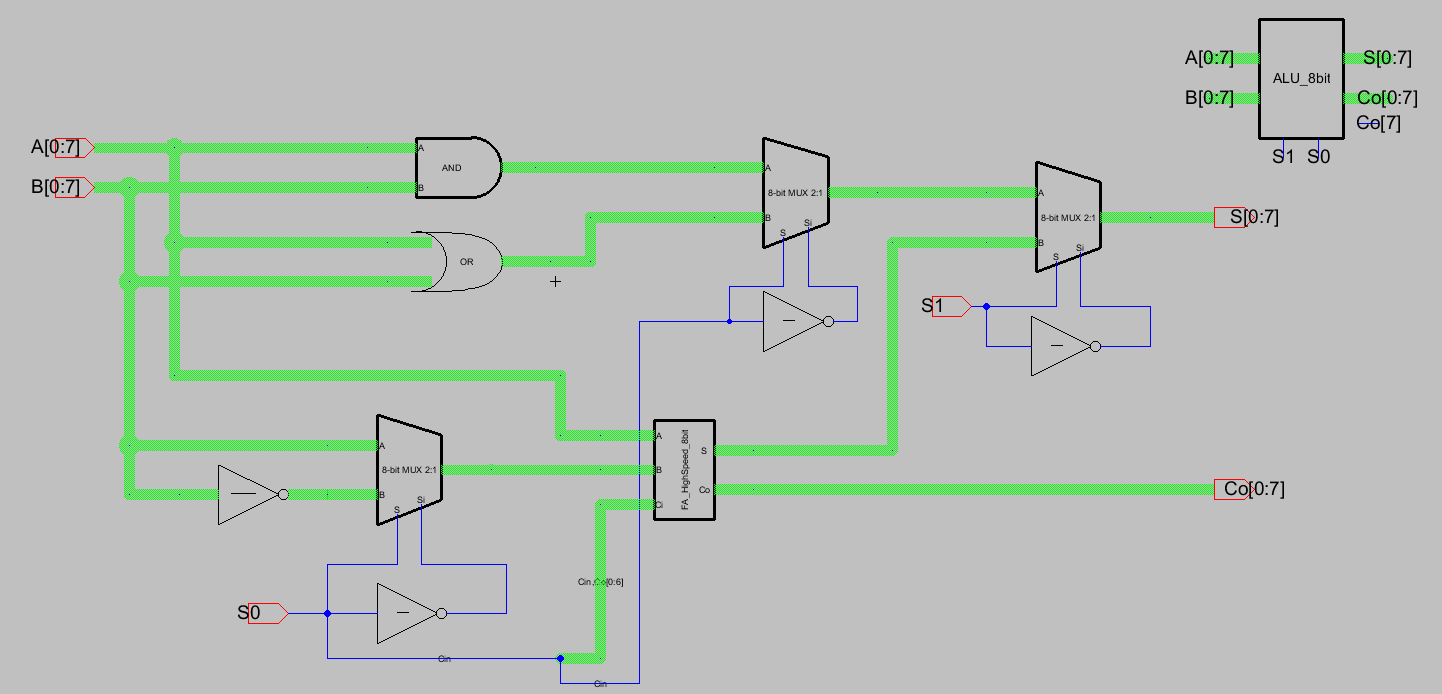Figure 3. Final schematic plus the icon for the simple 8-bit ALU.

Now that the schematic and icon were created, it was time to run some simulations to confirm that the ALU is functioning properly. I decided to stick with the four examples shown in the lab to verify the ALU's functionality.
The first simulation verified the AND gate's functionality by setting A to equal 1111 1111 and setting B to equal 0000 0000. The selection bits S1 and S0 were set to 00 and the LTSpice simulation performed. Figure 4 below shows the simulation schematic as well as the relevant spice code used to perform the simulation. Figure 5 shows the LTSpice simulation waveform plots of the 8-bit S output in addition to the 1-bit Co (carry out) output. If the ALU was functioning correctly then the expected output of 0 0000 0000 (Co, S[7:0]) should appear which was indeed verified in the LTSpice simulation.Figure 4. Simulation schematic for the first simulation performed. A is set to all HIGH while B is set to all LOW. When an AND operation is performed, the output should consist of all zeros.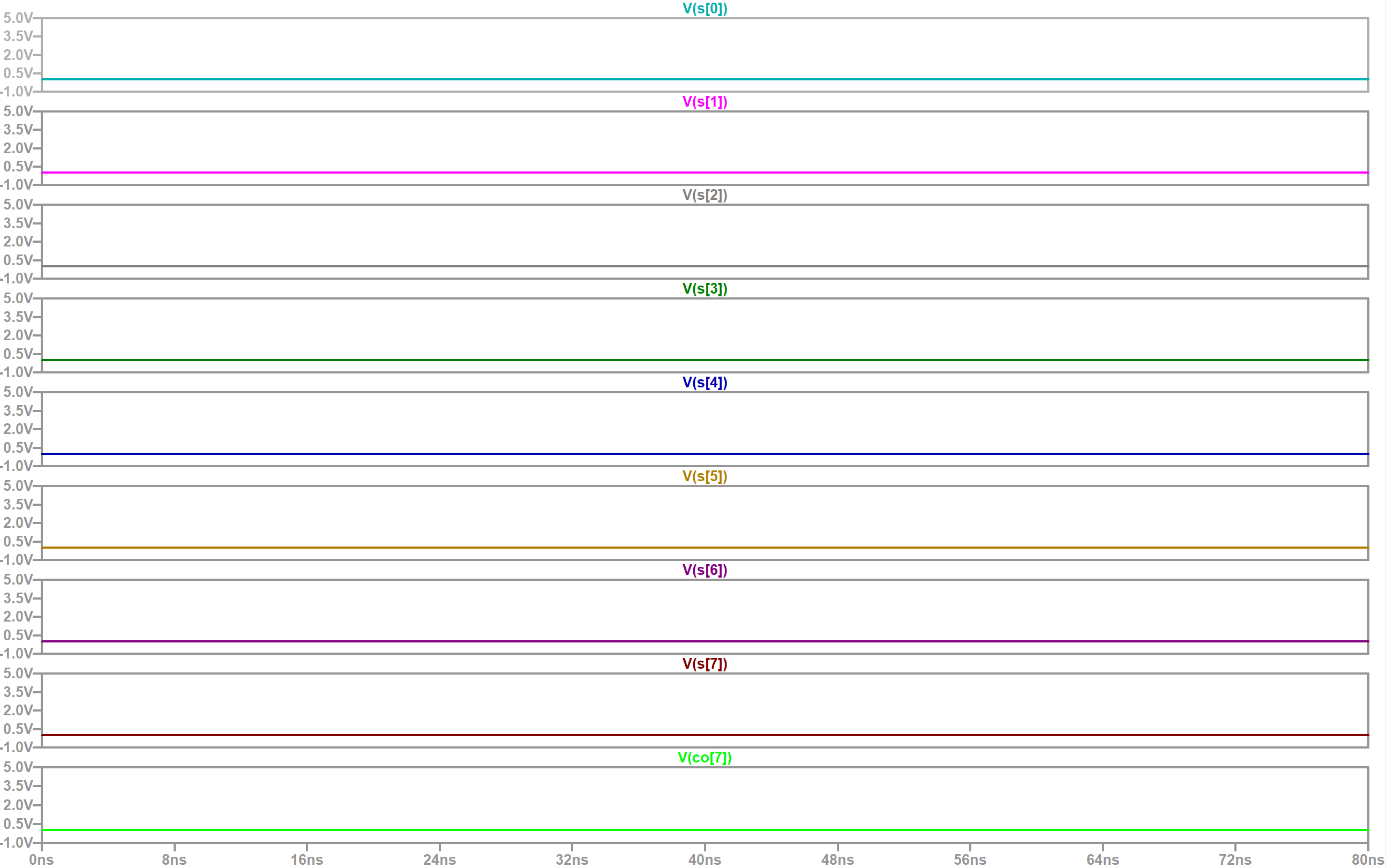Figure 5. LTSpice simulation results for the first simulation. The results match the expected outcome of simulation 1.

The next simulation to be run verified the OR gate's functionality. This was done by setting the selection bits to 01 while keeping the A and B inputs the same. Since A consists of all ones and B consists of all zeros, we expect the output to be all ones or 1 1111 1111 (which is Co and S[7:0]). This does match the LTSpice simulation results and so I moved onto the next simulation. Figure 6 shows the LTSpice simulation results for this test case but I decided not to display the simulation schematic since there was only a minor change was in the spice code.Figure 6. LTSpice simulation results for the second simulation. The results match the expected outcome of simulation 2.

The third simulation tested the addition operation. A was set to 1111 1111, B was set to 0000 0001, and the selection bits set to 10. The expected outcome of this addition was 1 0000 0000 which would be due to the carry functionality of the ALU operating correctly. The LTSpice simulation results match this expected outcome and so the addition operation was verified. Figure 7 displays the simulation schematic for this simulation since it differed from the previous simulations in multiple ways. Figure 8 displays the LTSpice simulation results for the third simulation.Figure 7. Simulation schematic for the third simulation, the addition test case.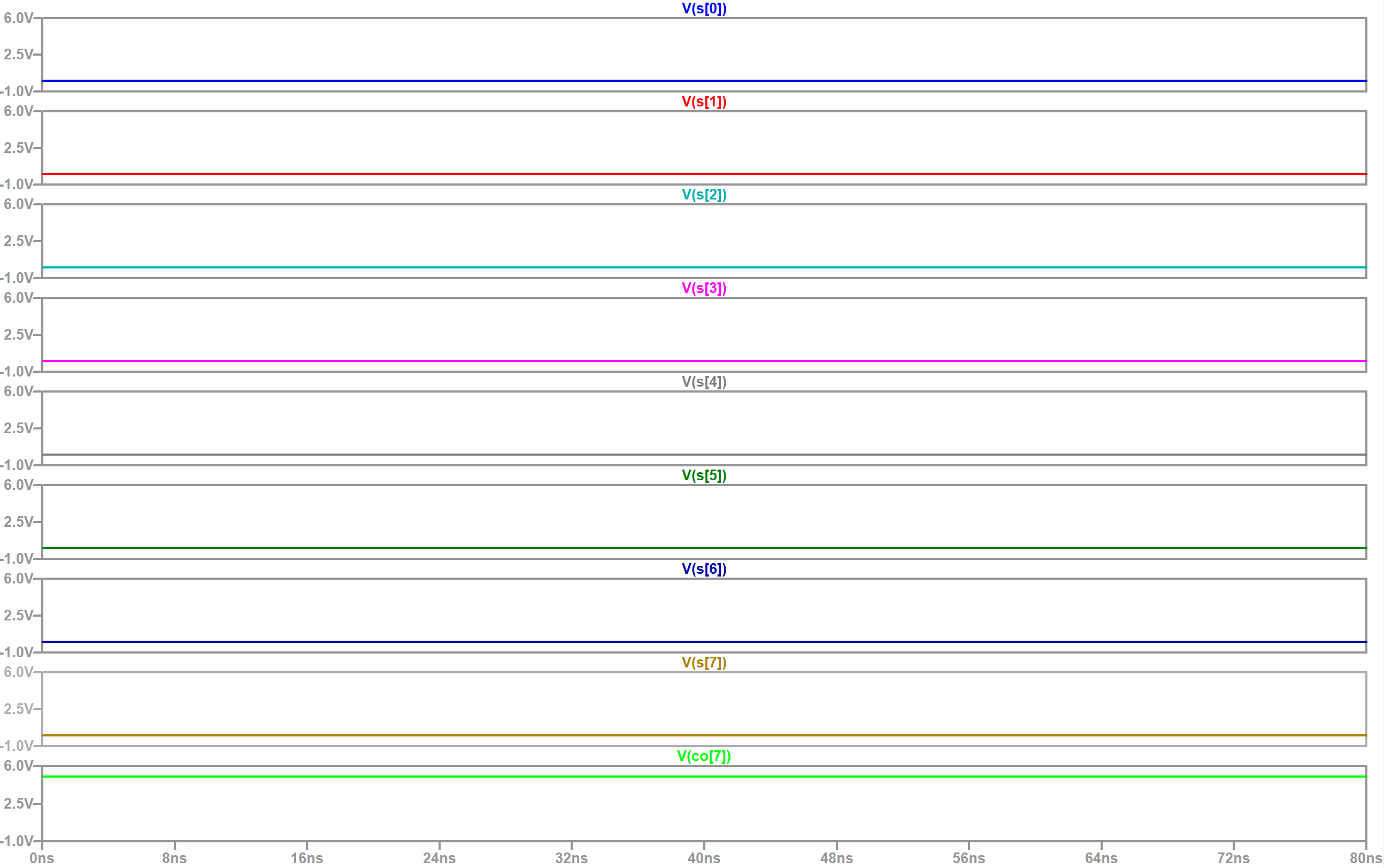Figure 8. LTSpice simulation results for the third simulation. The results match the expected outcome.

The final test case consisted of testing the subtraction operation. The A and B values were kept the same while the selection inputs, S1 and S0, were changed to 11. Due to the minor changes the simulation schematic was omitted. The expected outcome of 1111 1111 - 0000 0001 should be 1 1111 1110 (Co and S[7:0]) due to the 0000 0001 converting to the two's complement version (which is -1). The functionality was confirm as shown by the LTSpice simulation waveform shown below.Figure 9. LTSpice simulation results for the fourth simulation. The results match the expected outcome of 1 1111 1110.

Now that the functionality of the 8-bit ALU was verified, it was time to move onto creating the layout view for the ALU.

Task 2: Finish the layout of the ALU.
For this task we were given a video that gave us tips on wiring a large scale circuit by hand in addition to some direction given during lectures. A sample of what the finished ALU layout view could look like was also provided which was helpful as a starting point. The layout of the main pieces of the ALU like the AND and OR gates, the MUXes, and the full adder made sense to me so I tried to lay mine out similarly. I decided to focus on getting the wiring more compact and thinking about how to best use the metal layers. My finished layout is shown below in Figure 10 and you can click on it for a larger view of the layout. Figure 11 also displays the DRC and NCC check results which were great to see on the 2nd try.Figure 10. Final layout view of the simple 8-bit ALU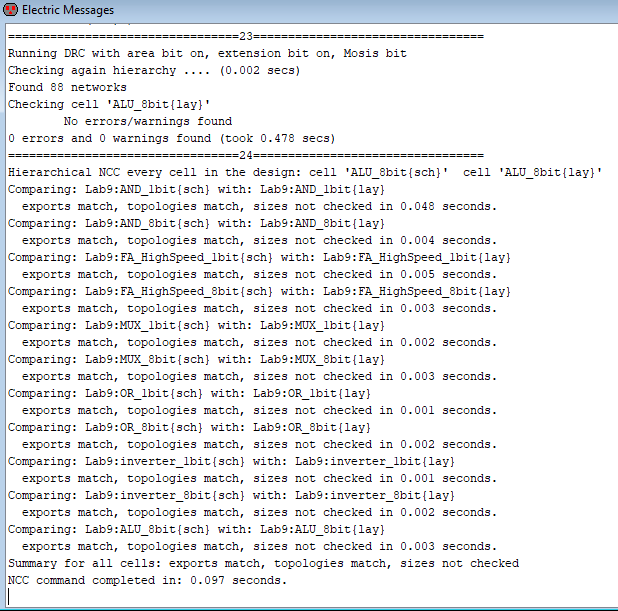Figure 11. DRC and NCC check results for the simple 8-bit ALU.

Discussion

I really enjoyed this lab because the design was something that could actually be useful to have on a PCB. I thought it was really cool to create a design that had multiple functions rather than the previous layouts that focused on doing one specific task like the logic gates or the MUX. It was also awesome to see that the layout design passed NCC only after two attempts. The first attempt gave me an error on the OR gate and that was when I realized I reversed the inputs so that B was going into the A port and vice versa. Thankfully, I was familiar with the error from a previous lab and I recognized right away that the simplest fix would be to correct the schematic view to have the inputs the same as the layout view. I also noticed that it was possible to only export the Co output instead of the Co[0:7] pins but when I attempted to do so, I couldn't pass NCC for some reason. I changed the original export on the schematic to be Co and deleted Co[0:6] on the layout view, but for whatever reason Electric VLSI wouldn't let the design pass NCC that way. So I ended up re-adding the exports for all the carry out outputs. I didn't enlarge the text on those exports though because they are only used in the full adder and do not have a pin out. The actual task of laying out the ALU design was a bit tedious, the worst was starting it because decisions made then would have to be dealt with later on. Towards the end I decided it'd be better if I wired the two inputs at the same time so I knew for sure how the rest of the wires would work, if they followed the correct pattern.